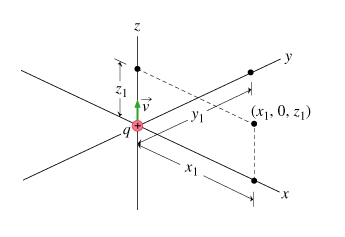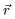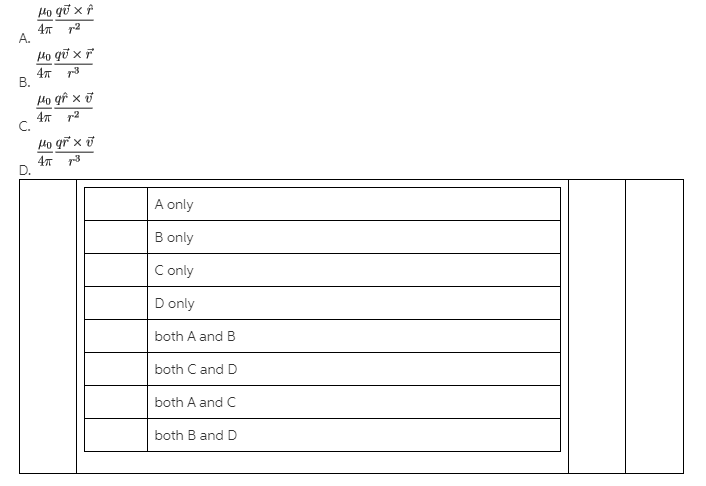# Problem: Magnetic Field near a Moving ChargeA particle with positive charge q is moving with speed v along the z axis toward positive z. At the time of this problem it is located at the origin, x=y=z=0. Your task is to find the magnetic field at various locations in the three-dimensional space around the moving charge. (Figure 1)Which of the following expressions gives the magnetic field at the point  due to the moving charge?

###### FREE Expert Solution

Biot-Savart law:

$\overline{)\stackrel{\mathbf{⇀}}{\mathbf{B}}{\mathbf{=}}\frac{{\mathbf{\mu }}_{\mathbf{0}}}{\mathbf{4}\mathbf{\pi }}{\mathbf{\int }}\frac{\stackrel{\mathbf{⇀}}{\mathbf{v}}\mathbf{×}\stackrel{\mathbf{^}}{\mathbf{r}}}{{\mathbf{r}}^{\mathbf{2}}}{\mathbf{d}}{\mathbf{q}}}$

Integrating Biot-Savart law:

$\begin{array}{rcl}\stackrel{\mathbf{⇀}}{\mathbf{B}}& \mathbf{=}& \frac{{\mathbf{\mu }}_{\mathbf{0}}}{\mathbf{4}\mathbf{\pi }}\mathbf{\int }\frac{\stackrel{\mathbf{⇀}}{\mathbf{v}}\mathbf{×}\stackrel{\mathbf{^}}{\mathbf{r}}}{{\mathbf{r}}^{\mathbf{2}}}{\mathbf{dq}}\\ & \mathbf{=}& \frac{{\mathbf{\mu }}_{\mathbf{0}}}{\mathbf{4}\mathbf{\pi }}\frac{\stackrel{\mathbf{⇀}}{\mathbf{v}}\mathbf{×}\stackrel{\mathbf{^}}{\mathbf{r}}}{{\mathbf{r}}^{\mathbf{2}}}\mathbf{\int }{\mathbf{dq}}\\ & \mathbf{=}& \frac{{\mathbf{\mu }}_{\mathbf{0}}}{\mathbf{4}\mathbf{\pi }}\frac{\mathbf{q}\stackrel{\mathbf{⇀}}{\mathbf{v}}\mathbf{×}\stackrel{\mathbf{^}}{\mathbf{r}}}{{\mathbf{r}}^{\mathbf{2}}}\end{array}$

97% (484 ratings)###### Problem DetailsMagnetic Field near a Moving Charge

A particle with positive charge q is moving with speed along the z axis toward positive z. At the time of this problem it is located at the origin, x=y=z=0. Your task is to find the magnetic field at various locations in the three-dimensional space around the moving charge. (Figure 1)
Which of the following expressions gives the magnetic field at the pointdue to the moving charge?